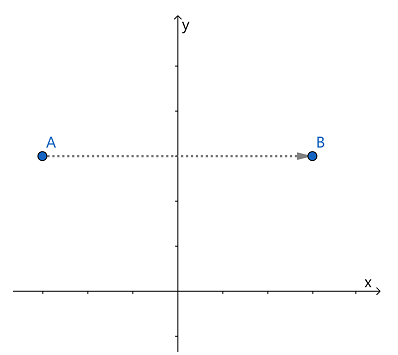安卓手机扫描二维码安装App﻿$\begin{cases} x'=-x \\ y'=y \end{cases}$﻿

﻿$\begin{cases} x'=-x +0y\\ y'=0x+y \end{cases}$﻿

﻿$\begin{bmatrix} -1 & 0 \\ 0 & 1 \end{bmatrix}$﻿

﻿$\begin{bmatrix} a & b \\ c & d \end{bmatrix}$﻿

﻿$x'=ax+by$﻿ ,

﻿$y'=cx+dy$﻿ .

﻿$P(x,y)$﻿ 关于直线 ﻿$Ax+By+C=0$﻿ 反射变换的坐标变换公式

﻿$\begin{cases} x'=x-2At, \\ y'=y-2Bt. \end{cases}$﻿

﻿$t=\dfrac{Ax+By+C}{A^2+B^2}$﻿

﻿$\begin{bmatrix} \dfrac{B^2-A^2}{A^2+B^2} & -\dfrac{2AB}{A^2+B^2} \\ -\dfrac{2AB}{A^2+B^2} & \dfrac{A^2-B^2}{A^2+B^2} \end{bmatrix}$﻿苹果手机扫描二维码安装App